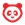cancel
Showing results for
Did you mean:

# What are Outcomes?

## What are Outcomes?

Outcomes allow the administration and faculty to track mastery in a course. Users can import Account, State, and Common Core Standards into an account and course.

## Account or Course Outcomes

Outcomes enables the administration and faculty to track students’ progress as measured by pedagogical goals or desired outcomes.

Assessments created to test student knowledge or to require students to demonstrate a specific skill resulting from a learning activity can be aligned to learning outcomes using rubrics (which can be used for grading simultaneously).

Grading student work automatically collects and compiles data on student progress for the Outcomes. The data is available for reporting to support teaching improvement, identify at-risk students, and support the accreditation process. This unified, streamlined approach dramatically reduces the amount of work required to implement Learning Outcomes through the intelligent reuse of assessment workflows in the grading process.

### When would I use Outcomes?

Use Outcomes to:

• Focus students' attention on the most important skills and activities in your course (find existing outcomes or create new ones)
• Align Quizzes and Assignments to different kinds of mastery
• Run reports at the account-level about student artifacts of learning mastery
• Assess student progress through calculation methods
• Track student progress on a learning outcome or overall in the Learning Mastery Gradebook
• Align accreditation or other core standards to programs of study, courses, or student assessments

## Calculation Methods

When users create an outcome, users can set one of four calculation methods used for student mastery: Decaying Average, n Number of Times, Most Recent Score, and Highest Score. Calculation methods are used in conjunction within the Learning Mastery Gradebook.

Calculation methods can be set when creating custom course outcomes (instructors) or creating custom account outcomes (admins).

Notes:

• For new Outcomes created in the Canvas interface, the default calculation is Decaying Average unless changed to another calculation.
• For new Outcomes created using the Outcomes API, the default calculation is set as Highest Score but can be modified with the appropriate parameters.
• If a user imports an outcome but does not have permission to modify the calculation method, the calculation method cannot be changed.
• Common Core outcomes are always calculated as Highest Score; however, the calculation method can be modified using the Outcomes API by any user with appropriate admin permissions.

### Decaying AverageThe Decaying Average calculation factors the average of all assessment items while weighing the most recent (current) submission to any assignment associated with this outcome at a higher percentage.

The term decaying average is traditionally used to describe a complex analysis: when an additional assessment is applied to an existing value, the prior decaying average is used to calculate the new decaying average. This method weights all assessments equally and calculates the average of learning over time. However, the Canvas method uses a simplified approach where Canvas calculates the average of all prior assessments and applies the value as a weighted percentage formula, instead of the alternative approach of simply using the prior decaying average.

By default, the Canvas decaying average is set at 65/35, meaning the current item is weighted at 65%, and the average of all other user scores is weighted at 35%. However, users can specify between 1% and 99% for the current percentage, and prior scores are weighted against the percentage difference. Note that the average is rounded to the next two decimals. If there is only one result, then the single score will be displayed.

For example, a student has four aligned items with scores of 4, 3, 2, and 5 (scores listed chronologically from oldest to most recent). If the current item is set to be weighted at 75% of mastery, prior scores are weighted at 25%:

• Current item score: 5
• Average of prior item scores: (4 + 3 + 2) / 3 = 3
• Calculated score: 5 (.75) + 3 (.25) = 3.75 + .75 = 4.5

### n Number of TimesThe n Number of Times calculation requires a specific number of times that mastery must be met or exceeded, and the number of aligned items that must be completed for calculation eligibility. Any scores that do not meet mastery are not part of the calculation.

Users can specify between 1 and 5 items required for mastery.

For example, a student is required to achieve mastery 2 times with a mastery score of 5. If the student has scores of 1, 3, 2, 4, 5, 3, and 6, only the scores of 5 and 6 would be part of the calculation.

• Average score of eligible items: 5 + 6 = 11
• Calculated score: 11 / 2 = 5.5

### Most Recent ScoreThe Most Recent Score calculation always selects the score for the most recent assessment item.

### Highest ScoreThe Highest Score calculation always selects the highest score for all assessment items.

Version history
Revision #:
7 of 7
Last update:
Wednesday
Updated by: